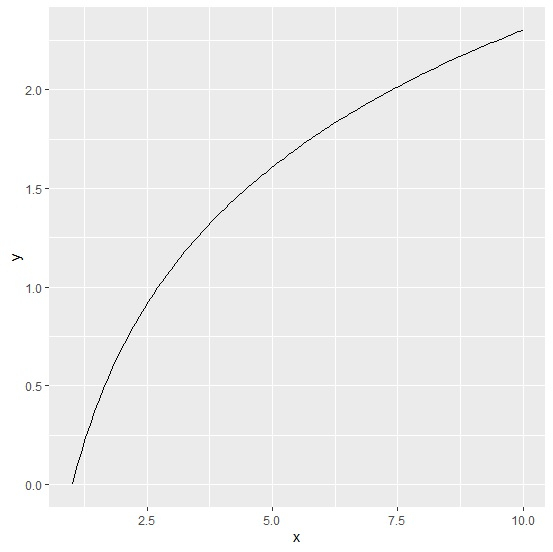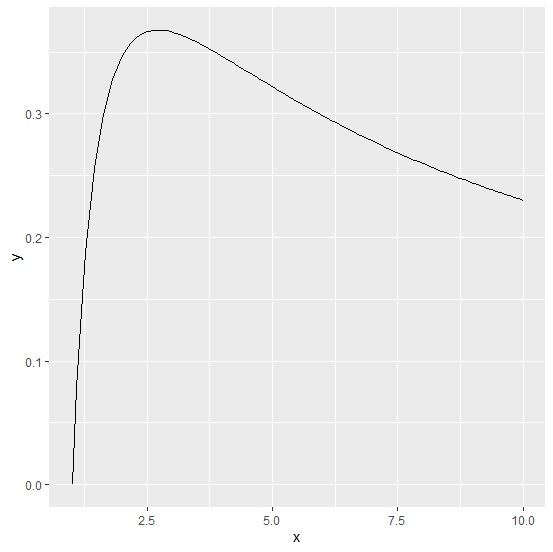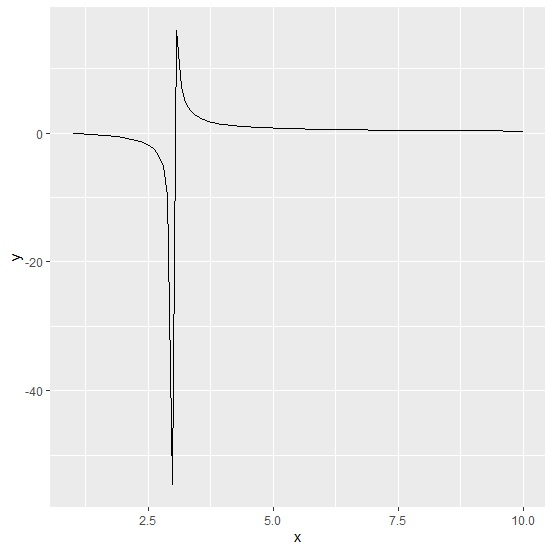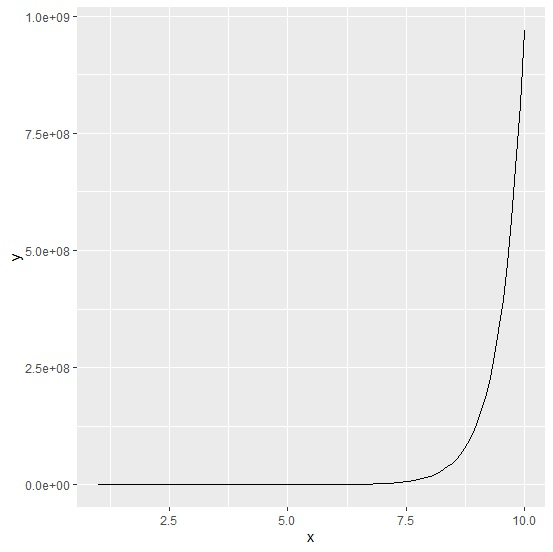# How to plot a function with ggplot2 in R?

Plotting a function is very easy with curve function but we can do it with ggplot2 as well. Since ggplot2 provides a better-looking plot, it is common to use it for plotting instead of other plotting functions. To plot a function, we should specify the function under stat_function in ggplot.

## Example

Consider the below data frame −

> x<-1:10
> df<-data.frame(x)

> library(ggplot2)

Plotting of functions is as shown below:

> ggplot(df,aes(x))+
+ stat_function(fun=function(x) log(x))> ggplot(df,aes(x))+
+ stat_function(fun=function(x) log(x)/x)

## Output> ggplot(df,aes(x))+
+ stat_function(fun=function(x) log(x)/(x-3))

## Output> ggplot(df,aes(x))+
+ stat_function(fun=function(x) (exp(x)^2)*2)

## OutputUpdated on: 12-Aug-2020

3K+ Views